## Binomial Coefficients and the Binomial Theorem

When a binomial is raised to whole number powers, the coefficients of the terms in the expansion form a pattern.These expressions exhibit many patterns:

• Each expansion has one more term than the power on the binomial.

• The sum of the exponents in each term in the expansion is the same as the power on the binomial.

• The powers on a in the expansion decrease by 1 with each successive term, while the powers on b increase by 1.

• The coefficients form a symmetrical pattern.

• Each coefficient entry below the second row is the sum of the closest pair of numbers in the line directly above it.

This triangular array is called Pascal's triangle, named after the French mathematician Blaise Pascal.

Pascal's triangle can be extended to find the coefficients for raising a binomial to any whole number exponent. This same array could be expressed using the factorial symbol, as shown in the following.In general,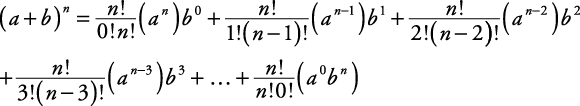The symbol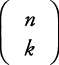, called the binomial coefficient, is defined as follows:Therefore,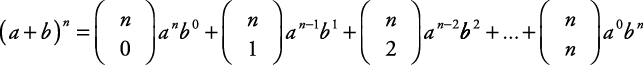This could be further condensed using sigma notation.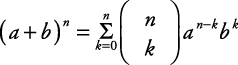This formula is known as the binomial theorem.

##### Example 1

Use the binomial theorem to express ( x + y) 7 in expanded form.Notice the following pattern:

•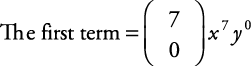••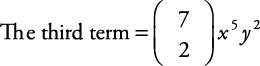In general, the kth term of any binomial expansion can be expressed as follows:##### Example 2

Find the tenth term of the expansion ( x + y) 13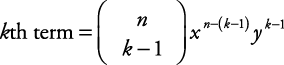Since n = 13 and k = 10,